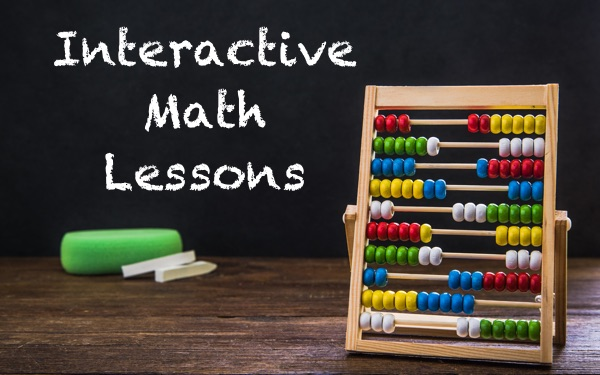# Interactive Math Lessons

Math is awesome! As a math teacher, you know this for a fact. Then why do students describe your favorite subject as “hard/boring/dry/all of the above”?

Maybe it’s because they’re too far away from it all, and that math is too abstract? Why not spice things up by adding some interactivity to your math course? Let them playfully explore the wonders of the world of numbers from the comfort of their own tablet or PC.

In this blog post, I’ll show how you can bring some interactivity to various math topics by creating your own, interactive, interesting, and fun widgets!

## Practice Basic Arithmetic

The youngest math students typically start by learning simple additions and subtractions, then moving on to multiplication and division.

Back in the old days, we used to have calculator toys to make practicing these simple exercises fun. These days, however, students can play fun arithmetic games straight from their own tablet or PC.

The Arithmetic Widget lets your students solve simple arithmetic problems, adapted to their level: as a teacher, you choose the kind of arithmetic exercises you want your students to do (additions, subtractions, multiplications, divisions, …), you choose the complexity (how many numbers should be added, how large can the numbers be, …); your students then get an interactive widget that lets them solve problems, and win rewards for each correct answer: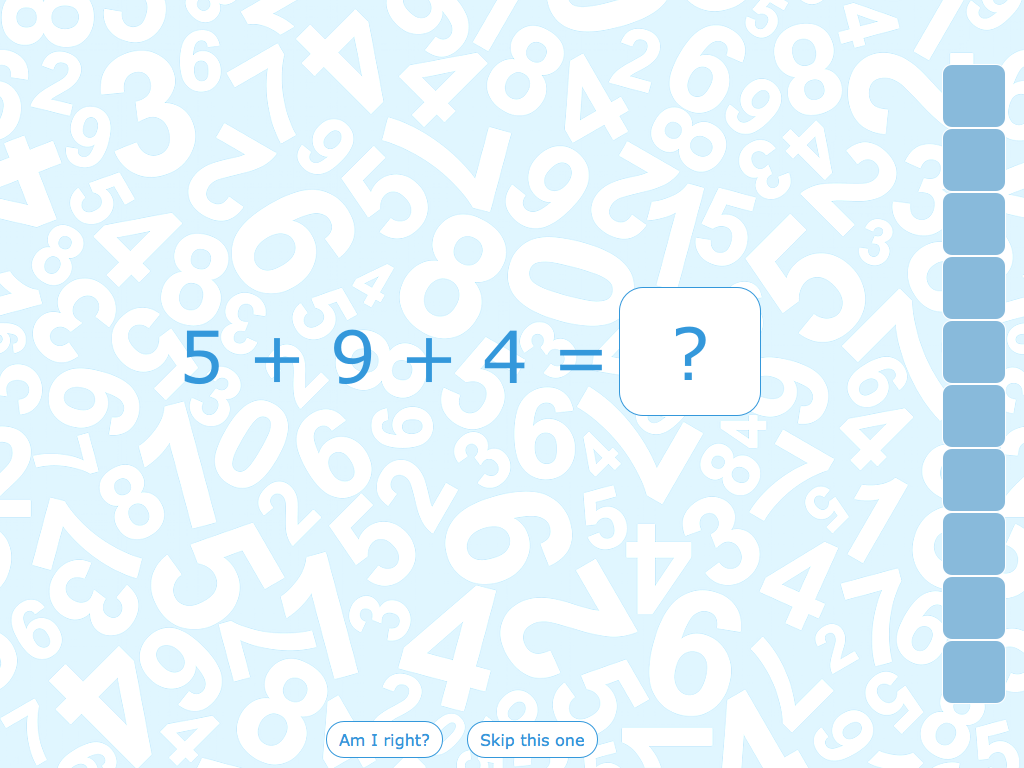## Interactive Graphs

A lot in math revolves around functions: linear functions, quadratic functions, trigonometric functions, … Knowing how these functions look like when plotted, and how they change when their coefficients change is key to understanding these functions and getting an intuitive feel for the math that uses them.

Because understanding function plots is so important, letting students interactively play with function visualizations is a very popular use of tablets or PCs in the classroom, and brings an otherwise dry topic to life. This is why the Active Plot widget is probably the most popular math widget in the BookWidgets library.

Creating your own interactive plot with the Active Plot widget is easy: all you have to do is enter the functions you want your students to learn about, and mark which parts of the function you want your students to interact with. For example, if we’re teaching about trigonometry, we can create an active plot for the sine and cosine functions: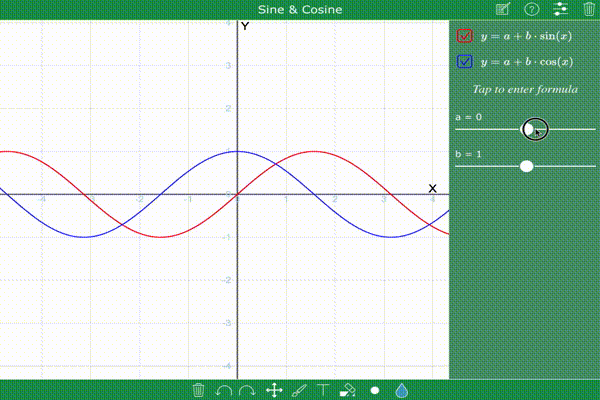By playing with the coefficient sliders, students get an understanding on how the coefficients affect amplitude and frequency.

You can take things even further: you can let students enter their own functions, or use drawing tools to draw on the plot (e.g. to indicate tangents). When they’re done, they can submit their answer to you, and you can grade them from within your dashboard.

## Probability Experiments

When teaching about probability in a statistics course, you can add some interactivity to your lesson by letting students do their own probability experiments.

To avoid coins flying around in class, you can easily do a coin flip experiment using the Randomness Widget: let students flip the coins a few times, make them count how many heads or tails they have, and let them come to the establish the fact that there’s a 50/50 chance of either.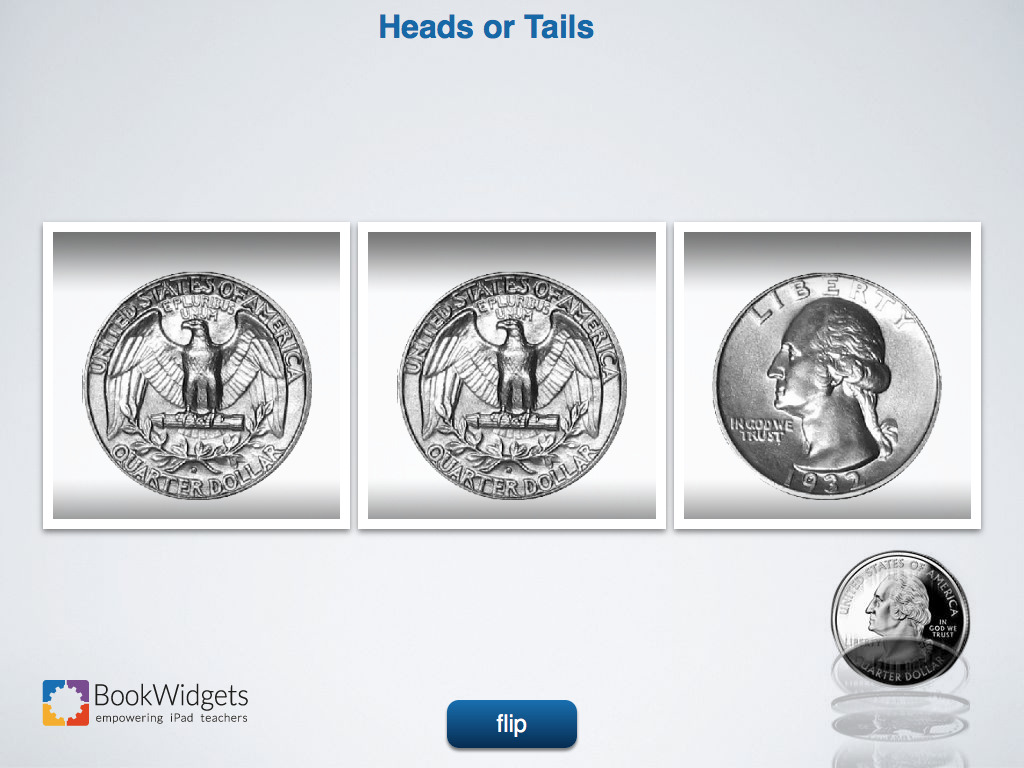Of course, you don’t have to use coin flips: you can use the Randomness widget to randomize anything, from numbers and symbols to your own custom images.

## Fun Classroom Exercise: Math Bingo

Bingo is the perfect classroom activity: each student gets a card with words or pictures in a random order; each time the teacher reads out a matching word, the student marks the word; the first student to have five words highlighted in a row yells ‘Bingo’, and wins.

There are lots of ways you can play Bingo in your math classroom. Here are just a few:

• Arithmetic Bingo: Read out sums, subtractions, divisions, and multiplications, and let the students find the answer within the numbers on their card. You can pair up students to help them find the numbers more quickly on their card.
• Fraction Bingo: Let the students find the fractions you read out within their squares. The card could contain the fractions explicitly written out (e.g. 2/3), or have a visual representation of the fraction such as pictures of pies with a number of pieces highlighted.
• Decimal Bingo: Put decimals on the card, and either call out the numbers literally, or as fractions.
• Roman Numeral Bingo: Fill the squares with roman numerals (XIV, MCM, L, III, …).
• Clock Reading Bingo: Fill the squares with pictures of clocks with hands in different positions, and read out the time.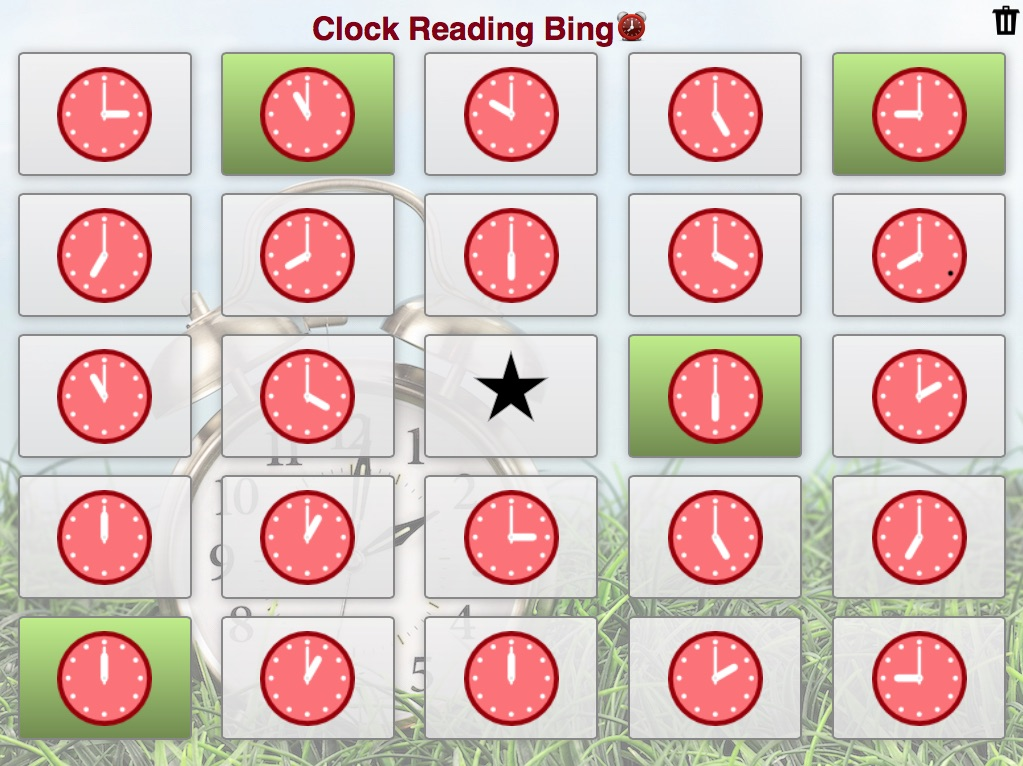## Solve Math Crossword Problems

The Crossword Widget is a fun way to learn about math terminology and definitions: you enter the clues or definition of a word, and students fill in the words in the crossword.

However, by playing with numbers, you can do some more out-of-the-ordinary crosswords as well. For example, you could create a list of arithmetic problems of a certain level, and let your students fill in the solution: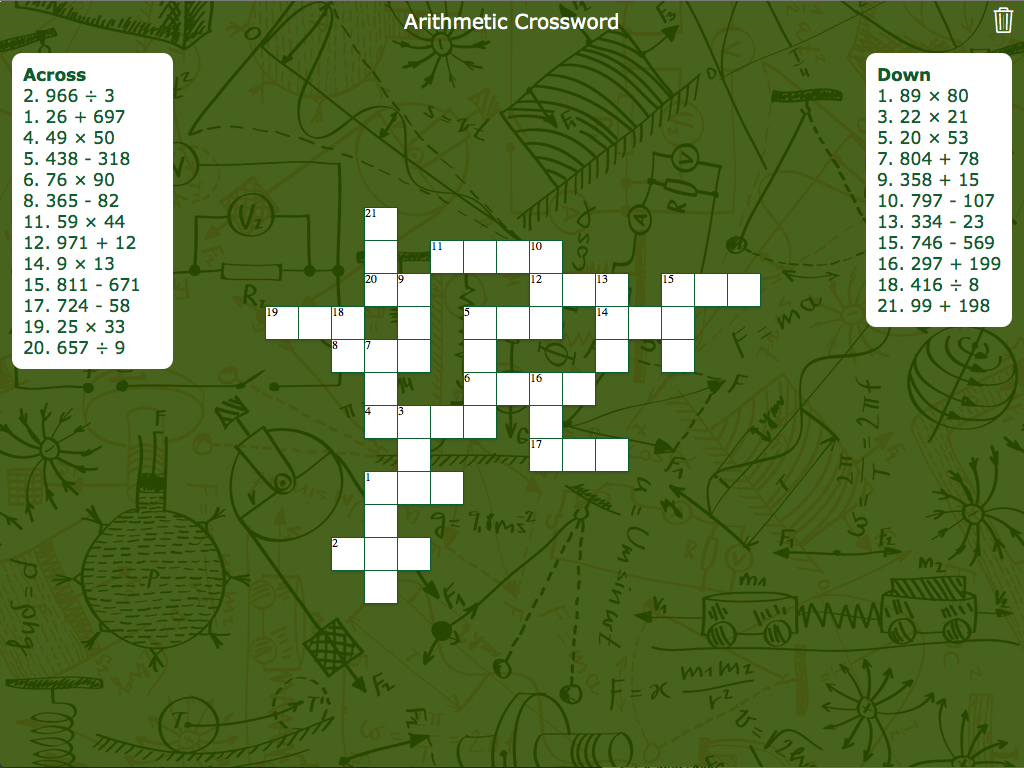This puts your students' brain cells at work, and gives a fun twist to simply writing down solutions to a long list of arithmetic problems. If arithmetic problems still sound too boring, you can make it more interesting by creating math problems (such as 12kg of apples cost €24. How much does 20kg of apples cost?) as clues of the crossword.

## Learn Math with Pair Matching Games

Another fun way to practice a math topic is by turning it into a pair matching game, where students have to find matching cards on the table. You can create card pairs for terms and their definitions, equations and their answers, …

The younger students can play pair matching games to get to learn their fractions: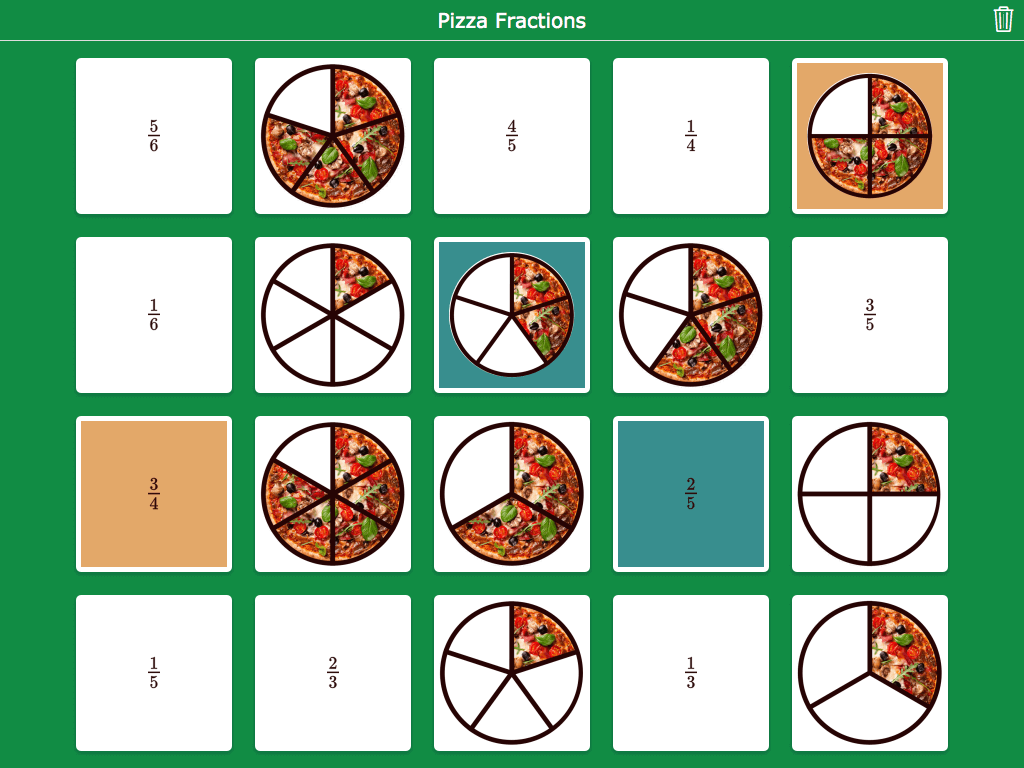## Study Math with Flash Cards

Although the most important part about studying math is understanding it, there’s always some parts you need to simply memorize. You can help your students study their definitions and formulae by using the Flash Card Widget to create interactive flash cards for your subject. For example, you can put pictures of geometrical shapes on one side, and enter the formula to compute their area or volume on the other side: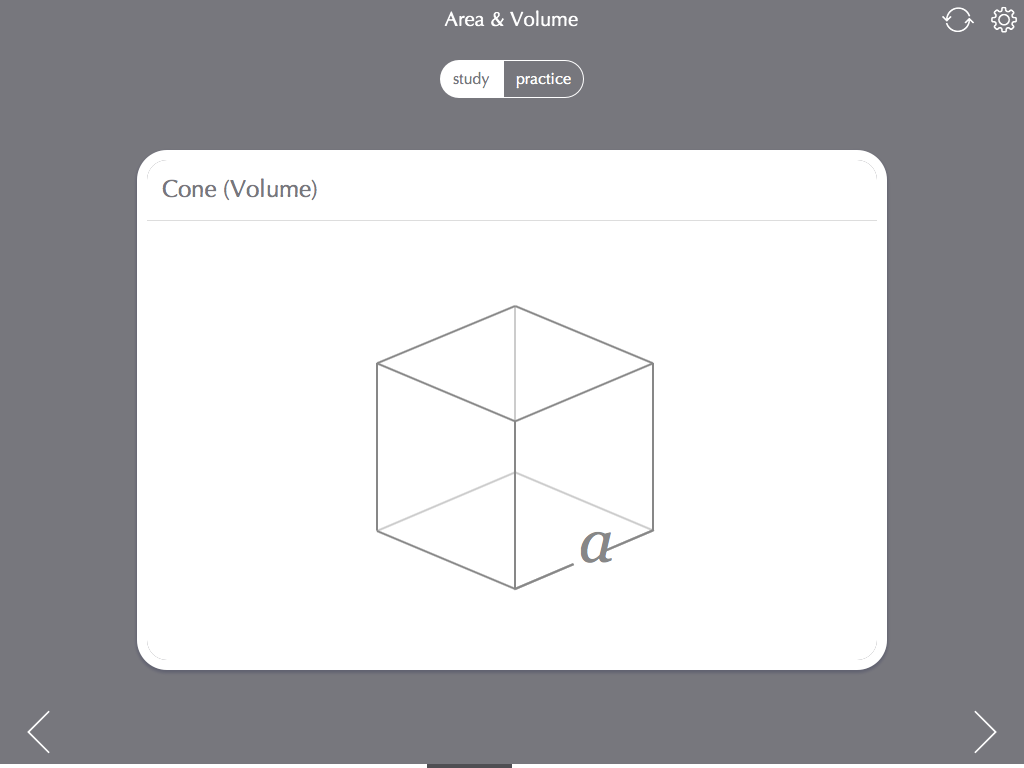When your students are done studying, they can go into practice mode to race against the clock, and focus on the cards they struggle with.

When your students are done studying math, the time has come to test their knowledge. The Quiz widget lets you do just that.

Besides generic question types such as multiple choice questions, annotating pictures, and whiteboard drawing questions, the Quiz widget offers question types tailored specifically to math. For example, students can answer questions with fractions, mixed numbers, or exponents: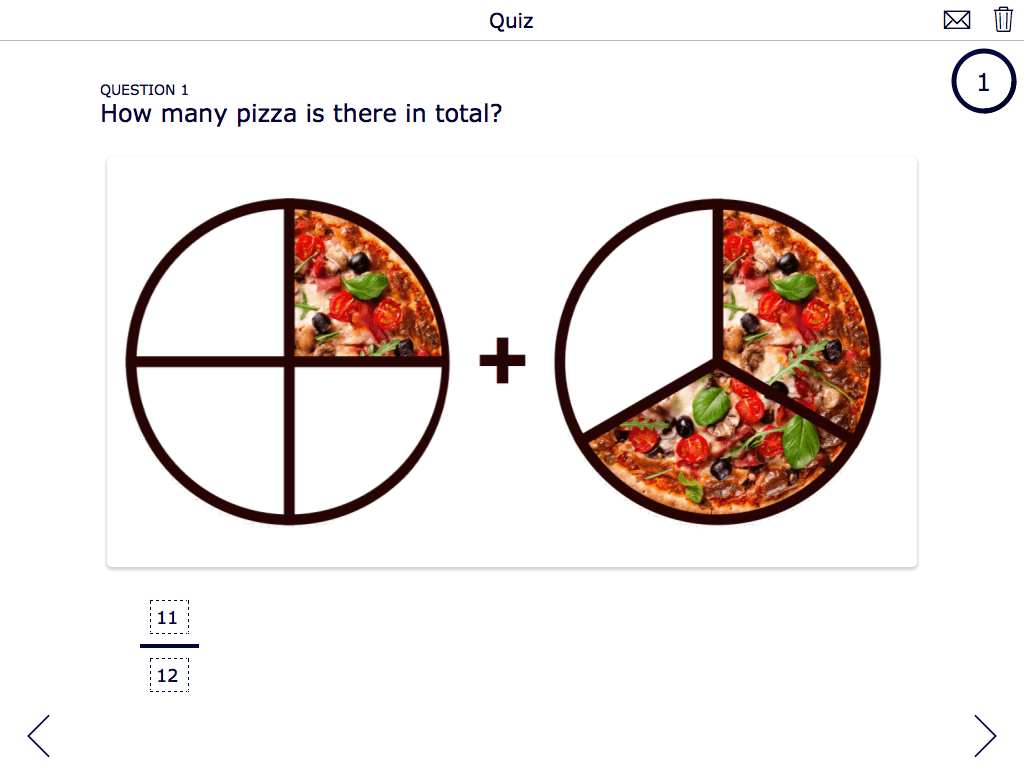Question types like these are automatically graded: when your student submits their answer to you, BookWidgets will automatically assign a score, so you don’t have to check the answer yourself. Needless to say, this can be a real time-saver during the already packed exam periods.

## Create your own Math widgets

I only showed a few possible uses of widgets to make your math class more interactive. Not only are there many more other widget types you can use in your math classroom (I didn’t even get to the Chart or Spreadsheet widget), but while reading this article, you probably already thought of your own application of some of the widgets I’ve shown earlier.

Ready to put your own ideas to work and make your math classroom more interactive? BookWidgets makes it easy to create your own math widgets: just hit the button below, sign up, and start creating your own math widgets with a free BookWidgets trial.

Remko Tronçon